Welcome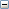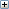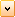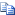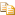ICreateTransformRotateAxis Method (IMathUtility)

Creates a new math transform matrix that represents the rotation by an angle about a vector positioned at a point.

# .NET Syntax

Visual Basic (Declaration)
```Function ICreateTransformRotateAxis( _
ByVal PointObjIn As MathPoint, _
ByVal VectorObjIn As MathVector, _
ByVal Angle As System.Double _
) As MathTransform```
Visual Basic (Usage)
```Dim instance As IMathUtility
Dim PointObjIn As MathPoint
Dim VectorObjIn As MathVector
Dim Angle As System.Double
Dim value As MathTransform

value = instance.ICreateTransformRotateAxis(PointObjIn, VectorObjIn, Angle)```
C#
```MathTransform ICreateTransformRotateAxis(
MathPoint PointObjIn,
MathVector VectorObjIn,
System.double Angle
)```
C++/CLI
```MathTransform^ ICreateTransformRotateAxis(
&   MathPoint^ PointObjIn,
&   MathVector^ VectorObjIn,
&   System.double Angle
) ```

#### Parameters

PointObjIn
Center point of the axis of the transform
VectorObjIn
Axis vector of the transform
Angle
Angle of rotation about the X axis vector

#### Return Value

Newly created math transform or NULL if the operation fails

# Availability

SOLIDWORKS 2001 FCS, Revision Number 9.0

Provide feedback on this topic

* Required

 *Email: Subject: Feedback on Help Topics Page: ICreateTransformRotateAxis Method (IMathUtility) *Comment: * I acknowledge I have read and I hereby accept the privacy policy under which my Personal Data will be used by Dassault Systèmes

Print Topic

Select the scope of content to print:

x

We have detected you are using a browser version older than Internet Explorer 7. For optimized display, we suggest upgrading your browser to Internet Explorer 7 or newer.

Never show this message again
x

Web Help Content Version: API Help (English only) 2018 SP05

To disable Web help from within SOLIDWORKS and use local help instead, click Help > Use SOLIDWORKS Web Help.

To report problems encountered with the Web help interface and search, contact your local support representative. To provide feedback on individual help topics, use the “Feedback on this topic” link on the individual topic page.